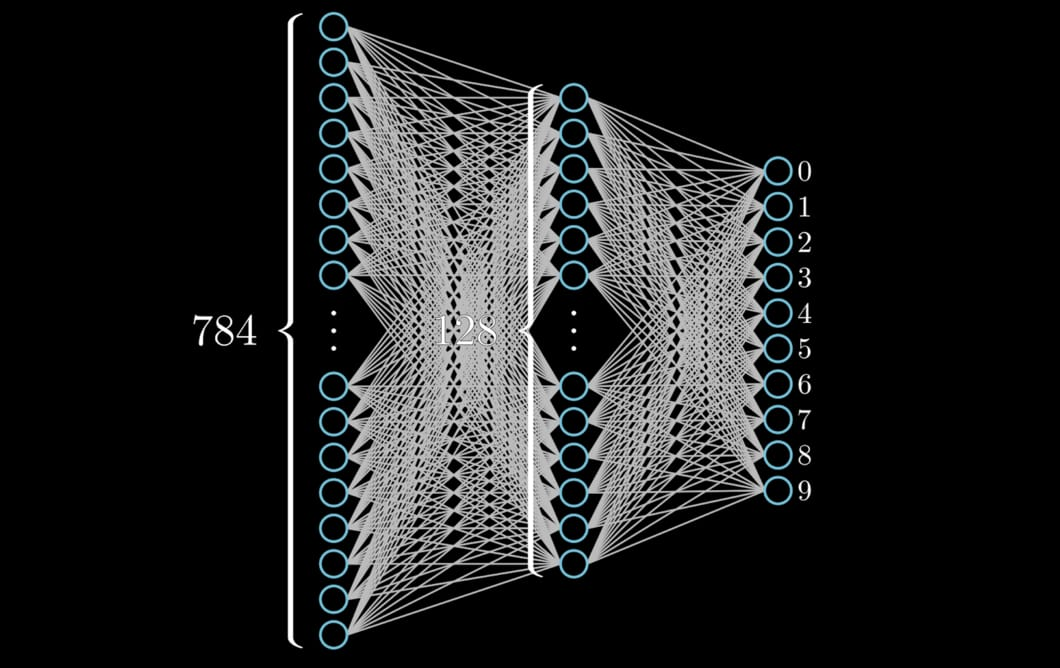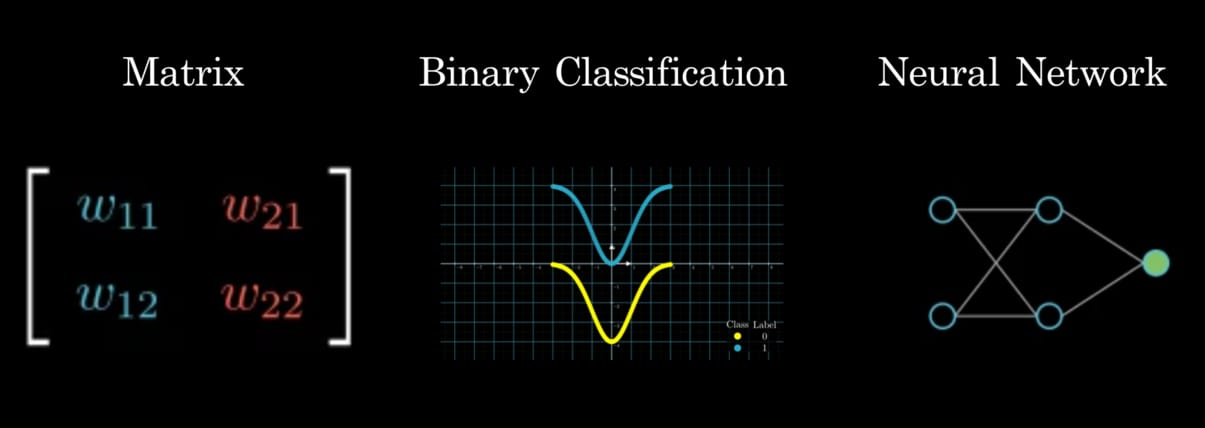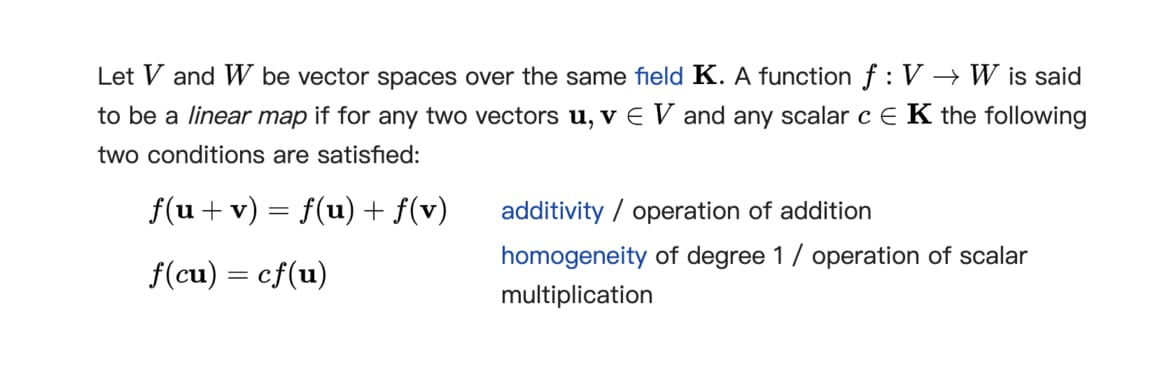（小提醒：因本文圖片與動畫皆為黑色背景，強烈推薦用左下按鈕以 Dark Mode 閱讀本文）

# 此例使用 TensorFlow，但各大深度學習框架的實現邏輯基本上類似
import tensorflow as tf

# 載入深度學習 Hello World: MNIST 數字 dataset
mnist = tf.keras.datasets.mnist
(x_train, y_train),(x_test, y_test) = mnist.load_data()
x_train, x_test = x_train / 255.0, x_test / 255.0

# 建立一個約有 10 萬個參數的「小型」神經網路
# 在現在模型參數動輒上千萬、上億的年代，此神經網路不算大
model = tf.keras.models.Sequential([
tf.keras.layers.Flatten(input_shape=(28, 28)),
tf.keras.layers.Dense(128, activation='relu'),
tf.keras.layers.Dropout(0.2),
tf.keras.layers.Dense(10, activation='softmax')
])

# 選擇損失函數、optimizer
loss='sparse_categorical_crossentropy',
metrics=['accuracy'])
# 訓練模型
model.fit(x_train, y_train, epochs=5)

# 訓練後的 NN 在測試集上可得到近 98% 正確辨識率
model.evaluate(x_test, y_test)

# 實際測試結果
# loss: 0.0750 - accuracy: 0.9763


## 一些有用的背景知識¶

1. 能讀懂文章開頭建立 NN 的 Python 程式碼
2. 了解線上課程都會教的超基本 NN 概念
3. 基本的線性代數概念如矩陣相乘、向量空間

## 深度學習框架操作容易，但你真的了解神經網路嗎？¶

# 在 TensorFlow 裡全連接層被稱作 Dense
# 因為這些層之間的神經元「緊密」連接
model = tf.keras.models.Sequential([
tf.keras.layers.Flatten(input_shape=(28, 28)),
tf.keras.layers.Dense(128, activation='relu'),
tf.keras.layers.Dropout(0.2),
tf.keras.layers.Dense(10, activation='softmax')
])model.summary()

Model: "sequential_8"
_________________________________________________________________
Layer (type)                 Output Shape              Param #
=================================================================
flatten_1 (Flatten)          (None, 784)               0
_________________________________________________________________
dense_9 (Dense)              (None, 128)               100480
_________________________________________________________________
dropout_1 (Dropout)          (None, 128)               0
_________________________________________________________________
dense_10 (Dense)             (None, 10)                1290
=================================================================
Total params: 101,770
Trainable params: 101,770
Non-trainable params: 0
_________________________________________________________________## 用二元分類連結神經網路 & 線性代數¶

• x 軸上藍色的 $\vec{i}$
• y 軸上紅色的 $\vec{j}$

─ Andrew Ng

\begin{align} f(x) & = f(\begin{bmatrix} x_{coord} \\ y_{coord} \end{bmatrix}) \\ & = f(\begin{bmatrix} x_{1} \\ x_{2} \end{bmatrix}) \\ & = y \end{align}

\begin{align} f(x) & = W x + b \\ & = \begin{bmatrix} w_{11} & w_{12} \end{bmatrix} x + b \\ & = \begin{bmatrix} w_{11} & w_{12} \end{bmatrix} \begin{bmatrix} x_{1} \\ x_{2} \end{bmatrix} + b \\ & = y \end{align}

1 × 2 矩陣運算與全連接層的對應關係

import tensorflow as tf
model = tf.keras.models.Sequential()
# 將 2 維 input  轉成 1 維 output
use_bias=False,
input_shape=(2, )))
model.summary()

Model: "sequential_6"
_________________________________________________________________
Layer (type)                 Output Shape              Param #
=================================================================
dense_6 (Dense)              (None, 1)                 2
=================================================================
Total params: 2
Trainable params: 2
Non-trainable params: 0
_________________________________________________________________


\begin{align} W = \begin{bmatrix} 1 & 5 \end{bmatrix} \\ x = \begin{bmatrix} 3 \\ 3 \end{bmatrix} \end{align}

1 × 2 矩陣運算與全連接層的對應關係（實際數值）

1. 線性轉換：$W$
2. 位移：$b$\begin{align} f(x) & = \begin{bmatrix} w_{11} & w_{12} \end{bmatrix} \begin{bmatrix} x_{1} \\ x_{2} \end{bmatrix} + b \\ & = \begin{bmatrix} 1 & 1 \end{bmatrix} \begin{bmatrix} x_{1} \\ x_{2} \end{bmatrix} + (-2) \end{align}

## 怎樣的神經網路才是好的分類器？¶

\begin{align} f(x) & = \begin{bmatrix} w_{11} & w_{12} \end{bmatrix} \begin{bmatrix} x_{1} \\ x_{2} \end{bmatrix} + b \\ & = \begin{bmatrix} 1 & 1 \end{bmatrix} \begin{bmatrix} x_{1} \\ x_{2} \end{bmatrix} + (-2) \end{align}

# 文章開頭的 MNIST 範例程式碼片段
# 定義一個能夠解決問題的模型架構
model = ...

# 設置學習實際參數所需的東西：損失函數、optimizer
loss='sparse_categorical_crossentropy',
metrics=['accuracy'])

# 訓練模型，取得最好的參數 theta
model.fit(x_train, y_train, epochs=5)

# 打完收工


\begin{align} W & = \begin{bmatrix} w_{11} & w_{12} \end{bmatrix} \\ & = \begin{bmatrix} 0.01 & 1.67 \end{bmatrix} \end{align}

## 由淺入深：解決看似不可能的分類任務¶

2-layers nn 解決困難雙曲線分類問題的過程

\begin{align} g(x) & = W_{2}(W_{1} x + b_{1}) + b_{2} \end{align}

\begin{align} \text{1-Layer NN} = f(x) & = W x + b \end{align}

2-Layers NN 裡頭的矩陣 $W_{1}$ 跟 1-Layer NN 裡頭的矩陣 $W$ 都代表著第一個 FC 做的線性轉換。但跟 1-Layer NN 不同的地方在於，$W_{1}$ 並不是直接把原始數據點 $x$ 轉換成一維的 $y$，而是先將 $x$ 轉到另個形式的二維表徵 $h$，接著再讓下一層 FC 將 $h$ 轉換至一維的 $y$。像是這種只存在輸入與輸出之間的表徵一般被稱作隱藏表徵（hidden representation），而生成這些表徵的 FC 層自然就被稱作隱藏層（hidden layer）。

2 × 2 矩陣 $w_{1}$ 的運算與全連接層之間的關係

• 一層 FC 對應到一矩陣運算（加偏差）
• 一個權重矩陣對應到一個線性轉換
• 一個線性轉換會對作用空間做旋轉伸縮

\begin{align} g(x) & = W_{2}(W_{1} x + b_{1}) + b_{2} \\ h(x) & = W_{2} relu(W_{1} x + b_{1}) + b_{2} \end{align}

## 結語：往下一站出發¶

1. 了解最基本的矩陣運算、神經網路以及兩者之間的緊密關係
2. 想像線性轉換如何對向量空間做旋轉、伸縮等基本轉換
3. 理解並想像一個 1-Layer NN 怎麼解決二元分類問題
4. 了解如何透過矩陣運算的形式定義一個神經網路架構 𝑓𝜃(𝑥)
5. 使用神經網路或是線性代數的角度來解讀 𝑓𝜃(𝑥) 的作用
6. 明白神經網路就是將原始數據 𝑥 進行一系列幾何轉換後輸出理想的 𝑦 的映射函數
7. 瞭解如何透過非線性的轉換，讓神經網路掌握更進階的能力
8. 自由地連結矩陣運算以及神經網路的概念並在兩者之間切換

2 × 3 矩陣與全連接層的對應關係